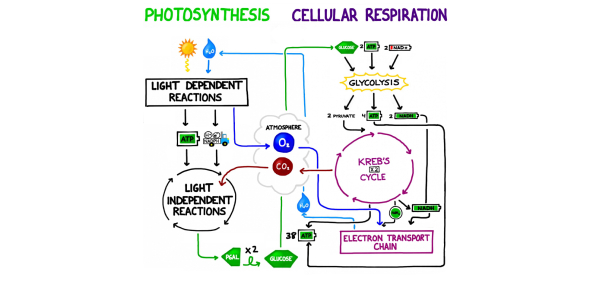A Quiz Over Photosynthesis And Cellular Respiration!

10 Questions | Total Attempts: 24630SettingsThis is a quiz over Photosynthesis and Cellular Respiration! Photosynthesis makes energy and respiration use it thus supporting life. What do you know about the process of photosynthesis and all the components needed for the process to go through swiftly? Take up the quiz below and get to know for sure. All the best, and remember to share the quiz with your friends.

• 1.
What is one of the reactants for cellular respiration?
• A.

Glucose

• B.

Water

• C.

Carbon dioxide

• D.

Energy

• 2.
What are the products for photosynthesis?
• A.

Sugar + glucose

• B.

Carbon dioxide

• C.

Sugar + oxygen

• D.

Water + energy

• 3.
During respiration, the reactants of photosynthesis are changed into:
• A.

H2O, CO2, and energy

• B.

C6H12O6 and O2

• C.

Energy and O2

• D.

C6H12O2 and H2O

• 4.
In cellular respiration what is the correct equation:
• A.

602+C6H12O6+energy -> 6CO2+6H2O

• B.

6CO2+6H2O+energy->6O2+c6H12O6

• C.

6O2+c6H12O6->6CO2+6H2O+energy

• D.

6O2+C6H12O6+energy->6CO2+6H2O

• 5.
How much ATP does aerobic respiration produce?
• A.

2 ATP

• B.

15 ATP

• C.

20 ATP

• D.

36 ATP

• 6.
Which is the correct equation for photosynthesis?
• A.

H2O + O2 -> energy+C6H12O6

• B.

CO2+H2O -> C6H12O6+O2

• C.

C6H12O6+energy -> H2O+O2

• D.

C6H12O6+O2 -> CO2+H2O

• 7.
What molecule does aerobic respiration use that anaerobic respiration is missing?
• A.

Oxygen

• B.

Glucose

• C.

Exercise

• D.

Light

• 8.
Cellular respiration occurs in which cell type?
• A.

Plant cells only

• B.

Animal cell only

• C.

Both plants and animal cell

• D.

All but plant cells

• 9.
What molecules belong in the box of the diagram?
• A.

1 transpiration; 2 respiration

• B.

1 chemosynthesis; 2 photosynthesis

• C.

1 respiration; 2 photosynthesis

• D.

1 photosynthesis; 2 respiration

• 10.
QIn cellular respiration energy can be released by breaking down which molecule?
• A.

Atp

• B.

Carbon doixide

• C.

Glucose

• D.

Water

Related TopicsBack to top